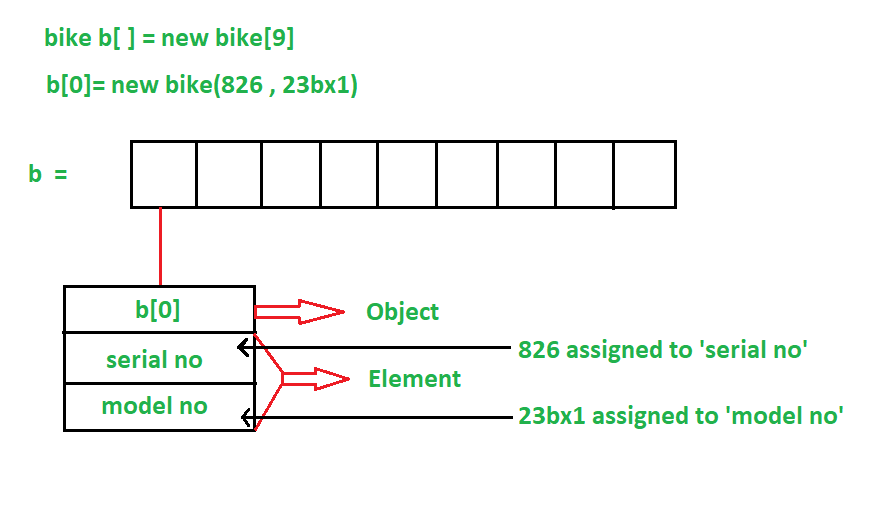Open in App
Not now

# How to Create Array of Objects in Java?

• Difficulty Level : Easy
• Last Updated : 02 Sep, 2022

Prerequisite – Different ways to create objects in Java

Java programming language is all about classes and objects as it is an object-oriented programming language. When we require a single object to store in our program we do it with a variable of type Object. But when we deal with numerous objects, then it is preferred to use an Array of Objects.

The array of Objects the name itself suggests that it stores an array of objects. Unlike the traditional array stores values like String, integer, Boolean, etc an Array of Objects stores objects that mean objects are stored as elements of an array. Note that when we say Array of Objects it is not the object itself that is stored in the array but the reference of the object.

Creating an Array Of Objects In Java –

An Array of Objects is created using the Object class, and we know Object class is the root class of all Classes.

We use the Class_Name followed by a square bracket [] then object reference name to create an Array of Objects.

`Class_Name[ ] objectArrayReference;`

Alternatively, we can also declare an Array of Objects as :

`Class_Name objectArrayReference[ ];`

Both the above declarations imply that objectArrayReference is an array of objects.

For example, if you have a class Student then we can create an array of Student objects as given below:

```Student[ ] studentObjects;
Or
Student studentObjects[];```

Instantiate the array of objects –

Syntax:

`Class_Name obj[ ]= new Class_Name[Array_Length];`

For example, if you have a class Student, and we want to declare and instantiate an array of Student objects with two objects/object references then it will be written as:

`Student[ ] studentObjects = new Student;`

And once an array of objects is instantiated like this, then the individual elements of the array of objects needs to be created using the new keyword.

The below figure shows the structure of an Array of Objects :Initializing Array Of Objects

Once the array of objects is instantiated, we need to initialize it with values.  We cannot initialize the array in the way we initialize with primitive types as it is different from an array of primitive types. In an array of objects, we have to initialize each element of array i.e. each object/object reference needs to be initialized.

Different ways to initialize the array of objects:

1. By using the constructors
2. By using a separate member method

1. By using the constructor:

At the time of creating actual objects, we can assign initial values to each of the objects by passing values to the constructor separately. Individual actual objects are created with their distinct values.

The below program shows how the array of objects is initialized using the constructor.

## Java

 `// Java program to demonstrate initializing``// an array of objects using constructor` `class` `GFG {` `    ``public` `static` `void` `main(String args[])``    ``{` `        ``// Declaring an array of student``        ``Student[] arr;` `        ``// Allocating memory for 2 objects``        ``// of type student``        ``arr = ``new` `Student[``2``];` `        ``// Initializing the first element``        ``// of the array``        ``arr[``0``] = ``new` `Student(``1701289270``, ``"Satyabrata"``);` `        ``// Initializing the second element``        ``// of the array``        ``arr[``1``] = ``new` `Student(``1701289219``, ``"Omm Prasad"``);` `        ``// Displaying the student data``        ``System.out.println(``            ``"Student data in student arr 0: "``);``        ``arr[``0``].display();` `        ``System.out.println(``            ``"Student data in student arr 1: "``);``        ``arr[``1``].display();``    ``}``}` `// Creating a student class with``// id and name as a attributes``class` `Student {` `    ``public` `int` `id;``    ``public` `String name;` `    ``// Student class constructor``    ``Student(``int` `id, String name)``    ``{``        ``this``.id = id;``        ``this``.name = name;``    ``}` `    ``// display() method to display``    ``// the student data``    ``public` `void` `display()``    ``{``        ``System.out.println(``"Student id is: "` `+ id + ``" "``                           ``+ ``"and Student name is: "``                           ``+ name);``        ``System.out.println();``    ``}``}`

Output

```Student data in student arr 0:
Student id is: 1701289270 and Student name is: Satyabrata

Student data in student arr 1:
Student id is: 1701289219 and Student name is: Omm Prasad```

2. By using a separate member method :

By using a separate member method also we can initialize objects. A member function of the respective class is created and that is used to assign the initial values to the objects.

The below program shows how the array of objects is initialized using a separate member method.

## Java

 `// Java program to demonstrate initializing``// an array of objects using a method` `class` `GFG {` `    ``public` `static` `void` `main(String args[])``    ``{` `        ``// Declaring an array of student``        ``Student[] arr;` `        ``// Allocating memory for 2 objects``        ``// of type student``        ``arr = ``new` `Student[``2``];` `        ``// Creating actual student objects``        ``arr[``0``] = ``new` `Student();``        ``arr[``1``] = ``new` `Student();` `        ``// Assigning data to student objects``        ``arr[``0``].setData(``1701289270``, ``"Satyabrata"``);``        ``arr[``1``].setData(``1701289219``, ``"Omm Prasad"``);` `        ``// Displaying the student data``        ``System.out.println(``            ``"Student data in student arr 0: "``);``        ``arr[``0``].display();` `        ``System.out.println(``            ``"Student data in student arr 1: "``);``        ``arr[``1``].display();``    ``}``}` `// Creating a Student class with``// id and name as a attributes``class` `Student {` `    ``public` `int` `id;``    ``public` `String name;` `    ``// Method to set the data to``    ``// student objects``    ``public` `void` `setData(``int` `id, String name)``    ``{``        ``this``.id = id;``        ``this``.name = name;``    ``}` `    ``// display() method to display``    ``// the student data``    ``public` `void` `display()``    ``{``        ``System.out.println(``"Student id is: "` `+ id + ``" "``                           ``+ ``"and Student name is: "``                           ``+ name);``        ``System.out.println();``    ``}``}`

Output

```Student data in student arr 0:
Student id is: 1701289270 and Student name is: Satyabrata

Student data in student arr 1:
Student id is: 1701289219 and Student name is: Omm Prasad```

Let’s see another example where an Array of Objects is declared with Initial Values:

Here declaration of an array of objects is done by adding initial values.

## Java

 `// Java program to demonstrate an array``// of objects is declared with initial values.` `class` `GFG {` `    ``public` `static` `void` `main(String args[])``    ``{``        ``// Creating an array of objects``        ``// declared with initial values``        ``Object[] javaObjectArray``            ``= { ``"Maruti"``, ``new` `Integer(``2019``), ``"Suzuki"``,``                ``new` `Integer(``2019``) };` `      ``// Printing the values``        ``System.out.println(javaObjectArray[``0``]);``        ``System.out.println(javaObjectArray[``1``]);``        ``System.out.println(javaObjectArray[``2``]);``        ``System.out.println(javaObjectArray[``3``]);``    ``}``}`

Output

```Maruti
2019
Suzuki
2019```

My Personal Notes arrow_drop_up